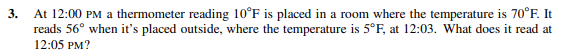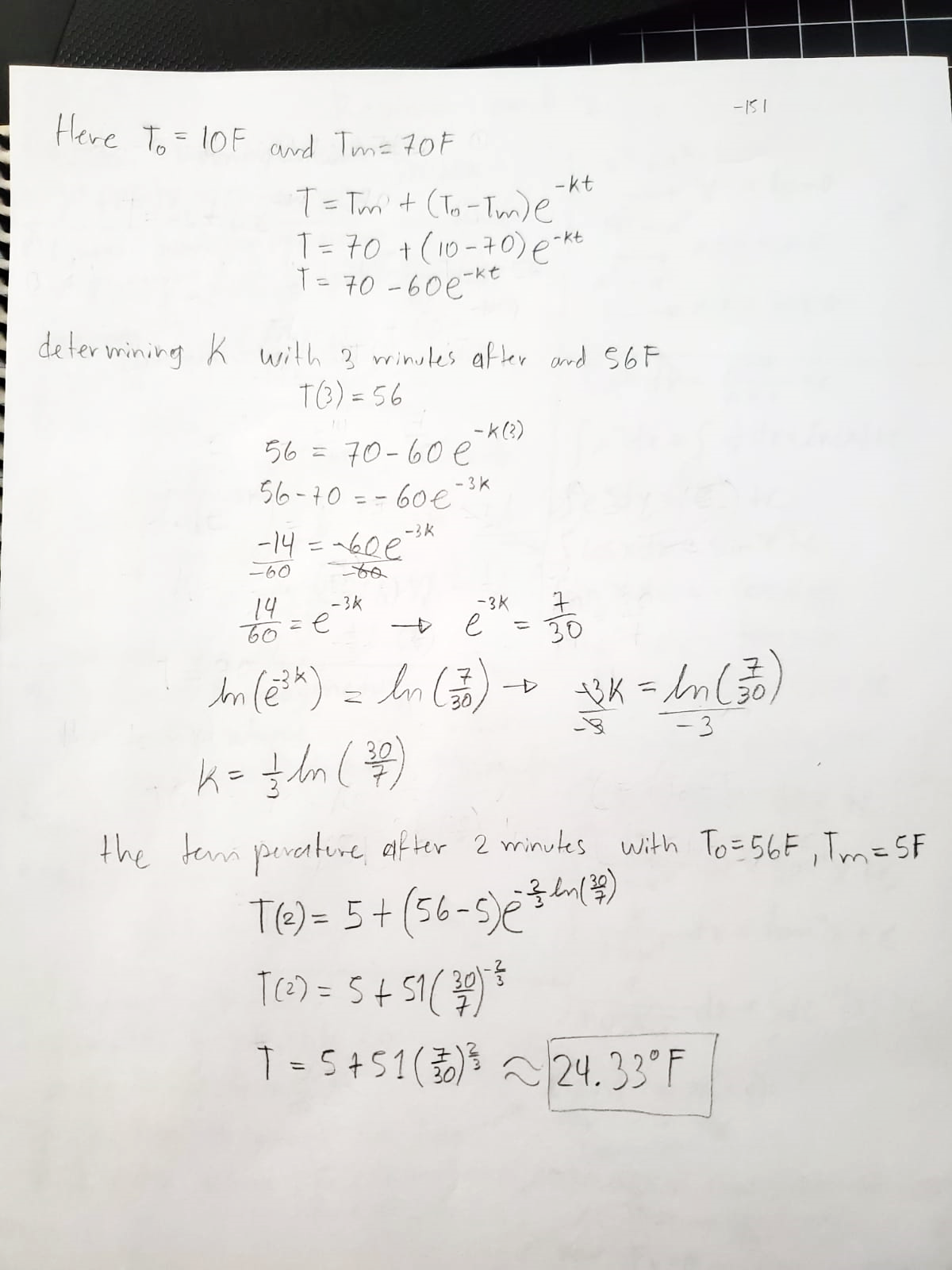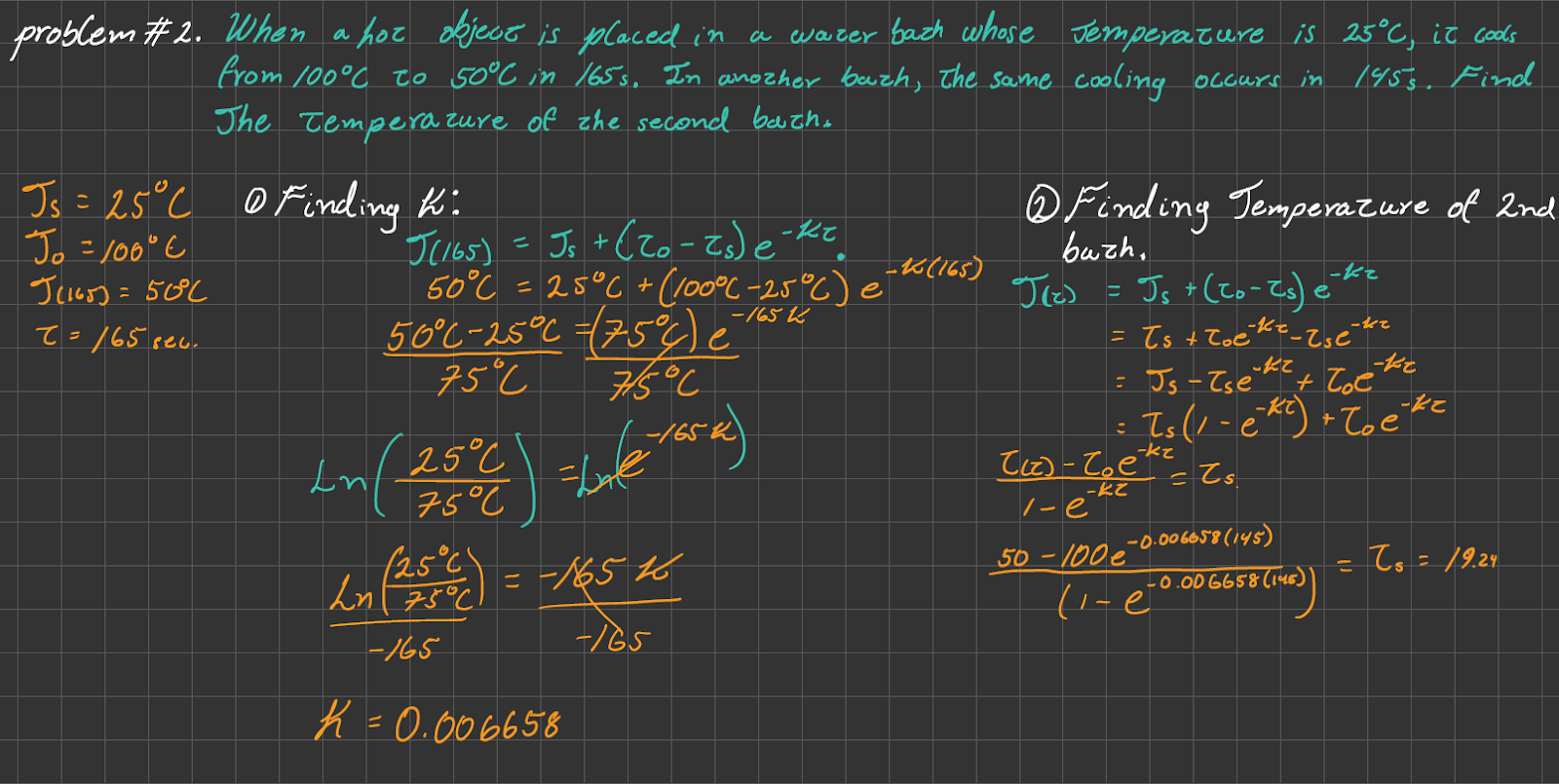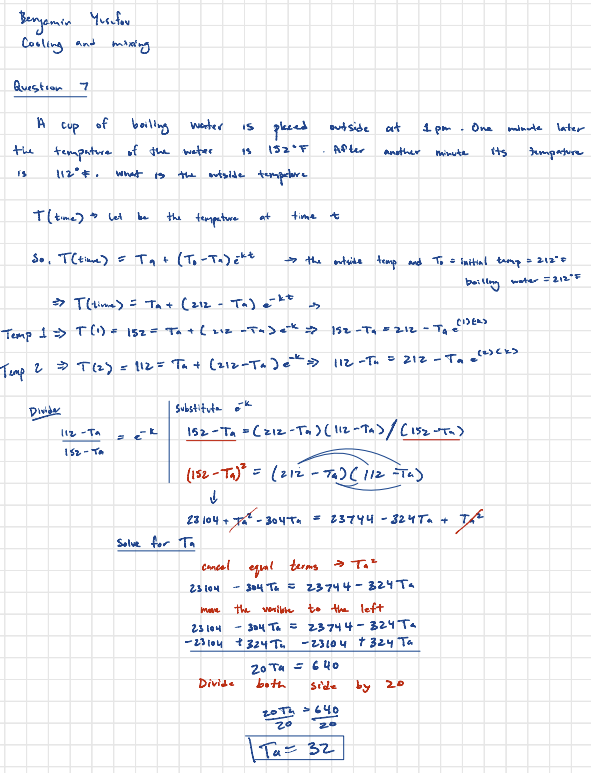Professor Kate Poirier | D772 | Spring 2023

Good day/evening classmates, in this post you will learn about 1 of the applications of First-Order Differential Equations. Specifically one of the topics found in Chapter 2, Section 4.2 of the Textbook ‘Elementary Differential Equations’ by author William F. Trench.

It talks about Cooling and Mixing problems. These kinds of problems are related to real world issues regarding the Mixture of certain components within a solution and the change of temperature of objects at a given time. Here the topic of Cooling is introduced and explained, examples are also provided.

# Lesson Summary

Newton’s Law of Cooling is a physical law that describes the behavior of the temperature of an object when it is in contact with a surrounding environment that is at a different temperature. This law was first formulated by Sir Isaac Newton in the late 17th century, and it has since been used to explain a wide range of phenomena in fields ranging from engineering to meteorology.

This equation can be used to model the cooling of an object that is initially hotter than its surrounding environment. As time passes, the temperature of the object will decrease until it reaches the same temperature as the surrounding environment.

As mentioned Newton’s law of cooling describes the rate at which an object cools as it loses heat to its surroundings. It states that the rate of cooling is proportional to the difference between the object’s temperature and the ambient temperature. This can be expressed as a first-order linear ordinary differential equation:

dT/dt = -k(T – T-a)

where T is the temperature of the object, T_a is the ambient temperature, t is time, and k is a constant that depends on the object’s physical properties and the properties of the surrounding medium.

The solution to this equation can be found using the separation of variables, which involves isolating the variables on opposite sides of the equation and integrating both sides. The result is an exponential decay function that describes the temperature of the object at any given time.

In conclusion, Newton’s Law of Cooling is a fundamental law of physics that describes the behavior of the temperature of an object in contact with a surrounding environment. By understanding this law and its applications, we can better understand the behavior of systems that involve heat transfer and design more effective cooling systems for a variety of applications.

# Example Problems

Contents

## Example #1

Here are some examples worked on by other colleagues, and videos to use as guides towards getting to know the solutions and how to work these kinds of problems.Made and solved by: Ricky Lin and Javier Bonilla

## Example #2Made and solved by: Nirvana Seeram and Jose Rodriguez

## Example #3Made and solved by: benjamin yusufov

## Example #4

Made and solved by: Javier Garcia and Damian Brathwaite

## Example #5

Made and solved by: Ameer and Kevin

## Example #6.

made and solved by: anushan thavasundaram and atta.

# Try It Out Yourself!

Here is a questions selected by one of out group members for you to try!

1.